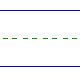Name: ______________________________________________ Date: _____________

Multiplication

Instructions: Multiply.

 7 x 5 =1 x 5 =3 x 9 =7 x 7 =3 x 4 =9 x 0 =6 x 4 =5 x 9 =3 x 2 =1 x 1 =9 x 2 =4 x 7 =6 x 5 =9 x 1 =9 x 8 =7 x 3 =4 x 0 =0 x 7 =